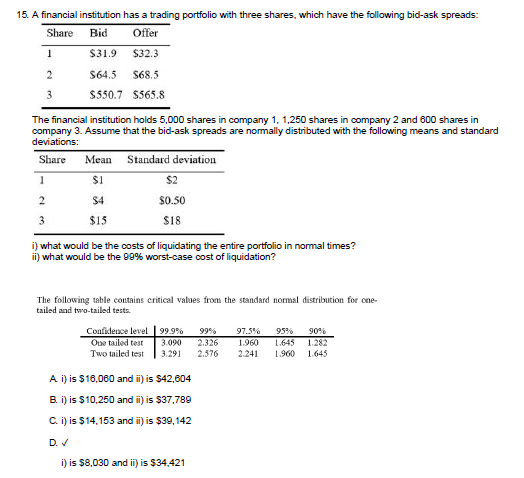# Costs of liquidation

Hello everyone,

Could anyone explain how to solve this case?For question i I come up with
(32,3+31,9)/25000=160.500
68,5+64,5)/2
1250=83.125
(565,8+550,7)/2*600=334.950
0,4/32,1 = 0,012
4/66=0,061
15,1/558,25=0,007
This times the capital gives
1.000
2.518,94
1.132,5
=4.651,4
Somehow I do not get to the right answer.
The second part is even harder.

If someone could help me I would be very grateful
Kind regards
Luc

i) Cost of liquidating the entire portfolio in normal times

Mid-price
Share 1: (31.9+32.3)/2 = 32.1
Share 2: (64.5+68.5)/2 = 66.5
Share 3: (550.7+565.8)/2 = 558.25

Share 1: (32.3-31.9) = 0.4
Share 2: (68.5-64.5) = 4.0
Share 3: (565.8-550.7) = 15.1

Mid-Market value
Share 1: \$32.1 \times 5,000 = \$160,500
Share 2: \$66.5 \times 1,250 = \$83,125
Share 3: \$558.25 \times 600 =\$334,950

= \$160,500 \times 0.012461/2 + \$83,125 \times 0.060150/2 + \$334,950 \times 0.027049/2 = \$8,030
= 5,000 \times \$0.4/2 + 1,250 \times \$4.0/2 + 600 \times \$15.1/2 1 Like ii) 99% worst-case cost of liquidation = 5,000 \times (\$1 + 2.326 \times \$2)/2 + 1,250 \times (\$4 + 2.326 \times \$0.50)/2 + 600 \times (\$15 + 2.326 \times \$18)/2 = \$34,417